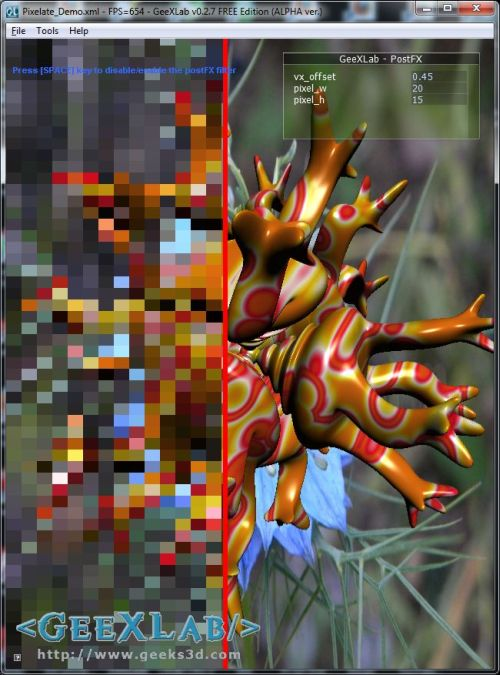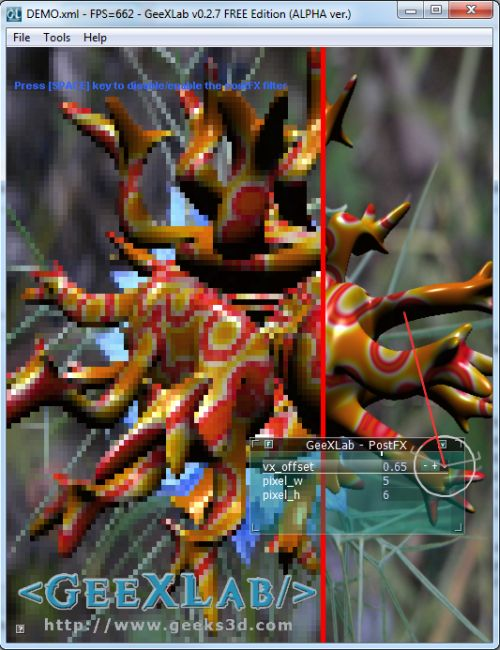# (Shader Library) Pixelation Post Processing Effect (GLSL)A pixelated Qoob mesh

This new shader, that you’ll find in Geeks3D’s shader library, shows how to pixelate the final rendering with a pixelation post processing GLSL shader.

Pixelation effect lowers the definition of an image and increases the size of the individual image pixels.

You can grab the GLSL shader and the GeeXLab demo here:

This demo requires GeeXLab 0.2.7 (for the Qoob mesh support) and does not use Python so you can use the version of GeeXLab without Python.

Unzip the source code somewhere, start GeeXLab and drop the file DEMO.xml in GeeXLab.Thanks to the tweak bar, you can modify in real time the different parameters

Language: OpenGL 2 – GLSL

Type: Post processing filter.

Inputs

• sceneTex (sampler2D): the final scene image.
• vx_offset (float): offset of the vertical red line
• rt_w (float): render target width (GeeXLab built-in uniform)
• rt_h (float): render target height (GeeXLab built-in uniform)
• pixel_w (float): width of a low resolution pixel
• pixel_h (float): height of a low resolution pixel

Ouputs: color buffer

```[Vertex_Shader]
void main(void)
{
gl_Position = ftransform();
gl_TexCoord = gl_MultiTexCoord0;
}
uniform sampler2D sceneTex; // 0
uniform float vx_offset;
uniform float rt_w; // GeeXLab built-in
uniform float rt_h; // GeeXLab built-in
uniform float pixel_w; // 15.0
uniform float pixel_h; // 10.0
void main()
{
vec2 uv = gl_TexCoord.xy;

vec3 tc = vec3(1.0, 0.0, 0.0);
if (uv.x < (vx_offset-0.005))
{
float dx = pixel_w*(1./rt_w);
float dy = pixel_h*(1./rt_h);
vec2 coord = vec2(dx*floor(uv.x/dx),
dy*floor(uv.y/dy));
tc = texture2D(sceneTex, coord).rgb;
}
else if (uv.x>=(vx_offset+0.005))
{
tc = texture2D(sceneTex, uv).rgb;
}
gl_FragColor = vec4(tc, 1.0);
}
```

## Credits

This pixelation shader is a modified version for GeeXLab of Agnius Vasiliauskas‘s original work.